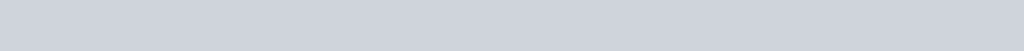ChemTalk

# Molecular Orbital Theory## Core Concepts

In this article, we learn about Molecular Orbital Theory, an important concept in quantum mechanics, as well as its application in understanding molecular bonds and antibonds.

## What is Molecular Orbital Theory?

Molecular orbital theory (MOT) is one of two fundamental bonding theories, along with valence bond theory (VBT), that help explain the formation of bonds between atoms. In particular, molecular orbital theory is useful in explaining how bonding chemically changes atomic behavior. Additionally, the theory accounts for phenomena not explained by valence bond theory, such as the paramagnetic nature of diatomic oxygen

At its most basic, molecular orbital theory states that the formation of bonds involves the mixing of atomic valence orbitals to form molecular orbitals. This differs from valence bond theory, which states that bonds form from shared valence electrons which stay in their atomic orbitals

## Forming a Molecular Orbital

In essence, a chemical bond involves the nuclei of two atoms attracted to one another’s electrons. As two nuclei approach one another, this attractive force increases. However, the two atoms also increasingly repel each other at shorter distances, due to the like charges of the nuclei and electrons. Ultimately, there exists some distance where the atoms are most attracted to each other before the repulsive forces dominate the interaction. When two atoms reach this perfect attractive distance, they are considered chemically bonded. This distance is denoted by the minimum (3) in the following graph:

Under molecular orbital theory, the valence orbitals of both atoms mix to form molecular orbitals. As the valence electrons fill these new molecular orbitals, they consequently behave differently than when occupying atomic orbitals. Thus, bonding under molecular orbital theory inherently changes the chemical behavior of the involved atoms.The electron orbitals of two atoms mixing to form a molecular bonding orbital.

According to the laws of quantum mechanics, the number of valence orbitals must remain constant for each atom. Thus, if the two s valence orbitals of two hydrogens mix, two molecular orbitals form: the sigma bonding orbital and sigma antibonding orbital. When electrons occupy the bonding orbital, the atoms’ potential energy drops as a sigma bond of mutual attraction forms. But what exactly is an antibonding orbital?

## Bonds and Antibonds

Spatially, for two nuclei to have attractive forces between valence electrons, those electrons must occupy some space between the two nuclei. This space is the bonding orbital.

We can also imagine that the nuclei approach with their electrons occupying the space on the other side of the nuclei. In this case, the attraction of the nuclei to their electrons draws the nuclei apart from each other, preventing a bond. This space is the antibonding orbital.

Further, remembering that electrons count as waves, like sound or light, we can think of a bond as a constructive interaction between the waves of the shared electrons. The combination of the atomic orbitals when they’re “in-phase”, meaning that the electrons both occupy the space between the nuclei, the interaction reinforces itself, forming a bond. However, if the electrons are “out-of-phase”, meaning that both do not occupy the space between the nuclei, the interaction is destructive, preventing further attractive forces between the nuclei.Antibonding (top) and bonding (bottom) s molecular orbitals. The dots correspond to nuclei or “nodes”/”antinodes” while the circles correspond to electron clouds.

## Differences between Valence Bond Theory and Molecular Orbital Theory

The following table summarizes the differences between valence bond theory and molecular orbital theory.

## Bonding Examples in Molecular Orbital Theory

Now that we understand both bonds and antibonds, let’s look at some examples.

#### H2 Bond Formation

When we have two neutrally-charged hydrogens, both atoms individually have one valence electron in the 1s orbital. As the hydrogens approach, their valence orbitals mix, forming the sigma bonding and antibonding orbitals. We can express these orbital formations using a molecular orbital diagram.

As mentioned before, the bonding orbital involves a drop in potential energy. Conversely, antibonding involves an increase in potential energy, as the atoms resist approaching. The molecular orbital diagram reflects these energy changes. Specifically, it displays the sigma bonding and antibonding orbitals respectively below and above the valence orbitals.

Because the atoms seek to lower their potential energy, their single valence electrons fill the sigma bonding orbital, forming a bond.

#### O2 Bond Formation

With two neutrally-charged oxygens, both atoms have six total valence electrons across four orbitals 2and 2p energy levels. Since all valence orbitals form molecular orbitals, the eight total valence orbitals between both molecules mix to form eight molecular orbitals. Like with hydrogen, the 2s orbitals form a sigma bonding and a sigma antibonding orbital. The two sets of three 2p orbitals form the same sigma orbitals, as well as two pi-bonding and antibonding orbitals.